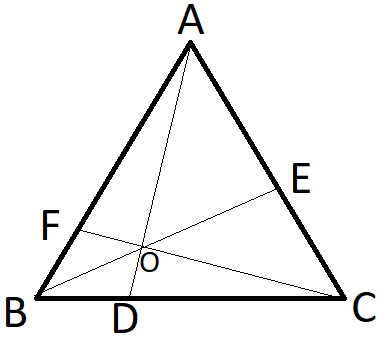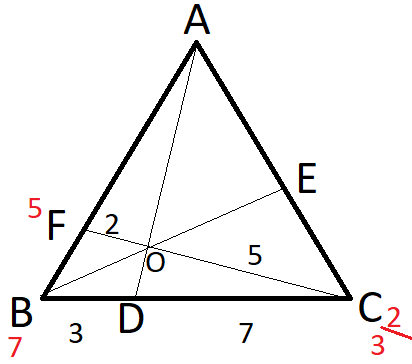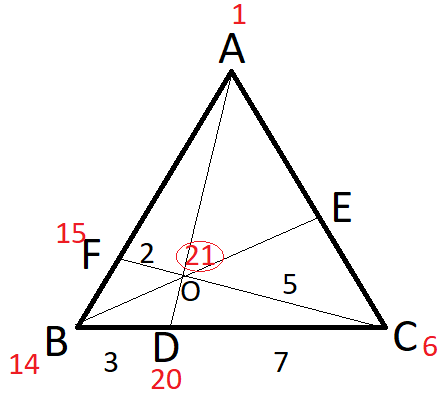Question of The Day20-04-2020

In the figure given below, if BD : DC = 3 : 7, OC : OF = 5 : 2, and AC = 14 cm, then what will be the value of EC?Correct Answer : a ) 2 cm

Explanation :

Applying Mass point theorem in the given figure we get,We consider the triangle composed of infinitesimally thin massless line segments to lie in a horizontal plane pivoted over a fulcrum placed at O. That means O is the center of mass for the triangle ABC.

Now, considering mass at point B equal to length of DC, i.e., 7 and mass at point C equal to the length of BD, i.e., 3.

Similarly, from line FC we can consider mass at point F = length of FO, i.e., 2 and mass at point C = length of OD, i.e., 2

Thus, we can say that we are getting two masses at point C, i.e., 3 and 2, but as at one point, there can be only one mass.

So, equating both the masses we can consider the mass at point C = LCM (2, 3) = 6

As we changed the mass at point C considering point C on line BC so, all the masses on line C have to be multiplied by a factor of 2

Also, we changed the mass at point C considering point C on line CF so, all the masses on line C have to be multiplied by a factor of 3

Thus, depicting all the masses on triangle we get,Therefore, we can say that masses at point A and C are 1 and 6 respectively

Thus, we can say that

$${Length\: of \:AE \over Length\:of\:EC}={Mass\:at\:point\: C \over Mass\: at\: point\: A}={6 \over 1}$$

$${Length\: of\: AE \over Length\:of\: EC}+1={6 \over 1}+1$$

$${Length\:of\:AC \over Length\:of\: EC}={7 \over 1}$$

$${14 \over Length\ of\ EC}={7 \over 1}$$

EC = 2 cm

Hence, (a) is the correct answer.

Such type of question is asked in various government exams like SSC CGL, SSC MTS, SSC CPO, SSC CHSL 2020, RRB JE, RRB NTPC, RRB GROUP D, RRB OFFICER SCALE-I, IBPS PO, IBPS SO, RRB Office Assistant, IBPS Clerk, RBI Assistant, IBPS RRB OFFICER SCALE 2&3, UPSC CDS etc.

Read Daily Current Affairs, Banking Awareness, Hindi Current Affairs, Word of the Day, and attempt free mock tests at PendulumEdu and boost your preparation for the actual exam.0